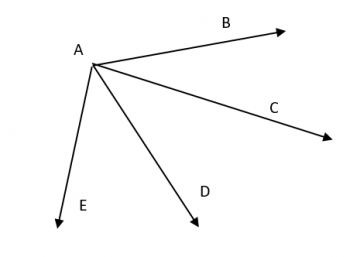Games
Problems
Go Pro!

# Angles and X

Pro Problems > Math > Geometry > Angles

## Angles and XIn the diagram above, the measure of angle EAC = 20 + 4x, the measure of angle BAD = 30 + 2x, and the measure of angle CAD = 10 + 6x. Find the measure of angle BAE.

Presentation mode
Problem by Mr. Twitchell

## Solution

In order to make it feasible for teachers to use these problems in their classwork, no solutions are publicly visible, so students cannot simply look up the answers. If you would like to view the solutions to these problems, you must have a Virtual Classroom subscription.Assign this problem

## Similar Problems

### Complementary and Supplementary

Angles A and B are complementary. Angles A and C are supplementary. The sum of the measures of B and C is 148 degrees. Find the measure of angle C.Like us on Facebook to get updates about new resources